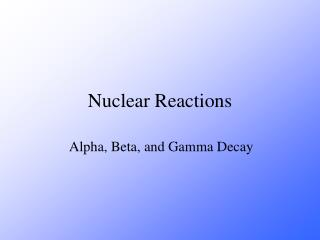DownloadDownload PresentationNuclear Reactions

# Nuclear Reactions

Télécharger la présentation## Nuclear Reactions

- - - - - - - - - - - - - - - - - - - - - - - - - - - E N D - - - - - - - - - - - - - - - - - - - - - - - - - - -
##### Presentation Transcript

1. Nuclear Reactions Alpha, Beta, and Gamma Decay

2. CS 4.2 State what is meant by alpha, beta and gamma decay of radionuclides. CS 4.3 Identify the processes occurring in nuclear reactions written in symbolic form.

3. The Atom The atom consists of two parts: 1. The nucleus which contains: protons neutrons 2. Orbiting electrons.

4. The Atom All matter is made up of elements (e.g. carbon, hydrogen, etc.). The smallest part of an element is called an atom. Atom of different elements contain different numbers of protons. The mass of an atom is almost entirely due to the number of protons and neutrons.

5. Mass number = number of protons + number of neutrons A X Element symbol Z Atomic number = number of protons

6. A X Z A = number of protons + number of neutrons Z = number of protons A – Z = number of neutrons Number of neutrons = Mass Number – Atomic Number

7. 238 235 U U 92 92 There are many types of uranium:

8. 238 235 U U 92 92 There are many types of uranium: Isotopes of any particular element contain the same number of protons, but different numbers of neutrons.

9. Most of the isotopes which occur naturally are stable. A few naturally occurring isotopes and all of the man-made isotopes are unstable. Unstable isotopes can become stable by releasing different types of particles. This process is called radioactive decay and the elements which undergo this process are called radioisotopes/radionuclides.

10. Radioactive Decay Radioactive decay results in the emission of either: • an alpha particle (a), • a beta particle (b), • or a gamma ray(g).

11. Alpha Decay An alpha particle is identical to that of a helium nucleus. It contains two protons and two neutrons.

12. A A - 4 X Y + Z Z - 2 4 He 2 Alpha Decay unstable atom alpha particle more stable atom

13. 226 222 Ra Rn 88 86 4 He 2 Alpha Decay

14. + A - 4 Y A X Z - 2 Z 4 4 226 222 He He Ra Rn + 2 2 88 86 Alpha Decay

15. 222 Rn + 86 A Y Z 222 218 4 4 4 Rn Po He He He + 86 84 2 2 2 Alpha Decay

16. A X + 230 Th Z 90 234 230 4 4 4 U Th He He He + 92 90 2 2 2 Alpha Decay

17. 230 230 Th Th + A Y 90 90 Z 226 4 4 4 Ra He He He + 88 2 2 2 Alpha Decay

18. A 218 X Po + 214 Pb 84 Z 82 214 4 4 4 Pb He He He + 82 2 2 2 Alpha Decay

19. Beta Decay A beta particle is a fast moving electron which is emitted from the nucleus of an atom undergoing radioactive decay. Beta decay occurs when a neutron changes into a proton and an electron.

20. Beta Decay As a result of beta decay, the nucleus has one less neutron, but one extra proton. The atomic number, Z, increases by 1 and the mass number, A, stays the same.

21. 218 218 Po At 84 85 0 b -1 Beta Decay

22. A A 0 0 b b X Y + Z Z + 1 -1 -1 218 218 Po Rn + 84 85 Beta Decay

23. 234 A 0 0 b b Th Y + 90 Z -1 -1 234 234 Th Pa + 90 91 Beta Decay

24. A 210 0 0 b b X Pb + Z 82 -1 -1 210 210 Tl Pb + 81 82 Beta Decay

25. 210 A 0 0 b b Bi Y + 83 Z -1 -1 210 210 Bi Po + 83 84 Beta Decay

26. A 214 0 0 b b X Bi + Z 83 -1 -1 214 214 Pb Bi + 82 83 Beta Decay

27. Gamma Decay Gamma rays are not charged particles like a and b particles. Gamma rays are electromagnetic radiation with high frequency. When atoms decay by emitting a or b particles to form a new atom, the nuclei of the new atom formed may still have too much energy to be completely stable. This excess energy is emitted as gamma rays (gamma ray photons have energies of ~ 1 x 10-12 J).